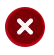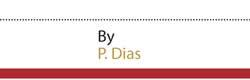# The “Z-Score” and the fate of A/L students

10 December 2020 01:44 am - 0     - {{hitsCtrl.values.hits}}Currently the University Grant Commission (UGC) uses the “Z-Score” as the basis for selecting students to universities. At G. C. E. (A/L) Examination, students sit for three subjects. Marks for each subject is given on a scale of 0-100. Thereafter, the Z-Score is calculated as follows.

Final Z-Score of the student=(Z1+Z2+Z3)/3
E.g.: Suppose if a student has obtained 60, 65 and 70 for three subjects. The mean mark of those subjects are 45, 40 and 50 respectively. Standard deviations are 15, 16 and 20 respectively.

The student’s Z-Score=[(60-45)/15+(65-40)/16+(70-50)/20] =[1+1.32+1]/3=3.5625/3=1.1875.History of the “Z-Score”
Before introducing the Z-Score, the aggregate mark (sum of the marks of three subjects) were used in selecting the students. When the Z-Score was introduced the students sat for four subjects at A/L. In physical or biological science streams. students sat for the same subject combination. Therefore, the use of the aggregate mark in selecting students had not been a problem. However, in Commerce and Arts streams, students sat for different subject combinations. Therefore, the use of aggregate was questionable. For example, students tend to select relatively easy subjects with the intention of simply getting a higher aggregate score. (Number of students who select Geography was decreasing since it was considered to be a relatively difficult subject both in Commerce and Arts streams). Z-Score method was proposed as a solution to this problem. When a subject is relatively easy that its mean becomes higher. In the Z-Score, the distance from the mean is considered in the numerator of the equation. Besides, the variability of the marks of the subject is also taken into account. Different subjects may have different means and different standard deviations. In translating the marks into Z-Score, the mean was changed to zero and the standard deviation was changed to 1.

Problems with the Z-score
Mean and standard deviations are not suitable measures of the centre and variability for asymmetric distributions. Marks of Mathematics subjects are not usually symmetric. The Z-Score is not suitable for subjects with a smaller number of candidates since one extreme value may seriously change the Z-Score. Subjects having low standard deviation contribute more to the final Z-Score than the subjects having higher standard deviations. For example, the standard deviation of Chemistry and Physics is around 15. However, it is around 20 for Combined Mathematics. Assume that mean mark for all these three subjects is 50 and student have scored 60 for each subject. The contribution to Z-Score from chemistry or physics is 10/15=0.67, and contribution from combined mathematics is 10/20=0.50.

Selection of students based on two parallel examinations
Usually, the National Institute of Education (NIE) changes syllabi of subjects once in every five years. With the syllabus changes, students at their first attempt sit for new syllabus and others sit for old syllabus for their examinations. As such, a single Z-Score cannot be used for a subject since they are sitting for two different papers. If we calculate Z-Scores for two groups separately they are not comparable since student groups are different. (Based on the results of the first attempt, some get the admission to universities and they do not sit for the second attempt. Others who got very low results might not sit for the exam again. Therefore, it is not reasonable to compare the Z-Scores calculated for the two different groups.) Therefore, the solution suggested here is to consider the average proportion of students selected in each attempt during the last five years. It seems reasonable but not a perfect solution since the proportions are varying from year to year.

Alternative solutions
Though syllabus revisions are taken place once in every five years, usually not more than 10-20 per cent of the contents of the syllabi are changed for each subject. Therefore, the best thing is to prepare a single paper for each subject having alternative questions for the part of the syllabus changed. Then it is possible to calculate the single Z-Score.

Alternatives for Z-Score
The average of percentiles of the students for each subject can be considered as an alternative to the Z-Score. Percentile means the percentage of students below or equal to the mark obtained by the student. This provides solutions to two problems namely the inappropriateness of mean and standard deviation and influence of extreme values. However, the disadvantage of this method is that the mark difference is not taken into account, only the relative position is considered.

The other possibility is that use the aggregate for students who are following the same subject combination. For example, this can be implemented easily for Engineering, Medicine, Biological Science, Physical Science, Engineering Technology and Biotechnology streams. This is useful for students who are very keen on a particular subject. For example, one may be very good at Combined Mathematics but may be somewhat weak in Chemistry. In such a case, he gets the opportunity to get into the university and become a Mathematician. Under Z-Score he may not get that opportunity.

The writer is a Senior Lecturer Department of
Statistics at the University of Sri Jayewardenepura

Order Gifts to Sri Lanka. See Kapruka's top selling online shopping categories such as Toys, Vegetables, Grocery, Flowers, Birthday Cakes, Fruits, Chocolates, Clothing and Electronics. Also see Kapruka's unique online services such as Money Remittence,News, Courier/Delivery, Food Delivery and over 700 top brands. Also get products from Amazon & Ebay via Kapruka Gloabal Shop into Sri Lanka.

##